• 最大熵模型中的对数似然函数的解释 最近在学习最大熵模型，看到极大似然估计这部分，没有看明白条件概率分布p(y|x)p(y|x)p(y|x)的对数似然函数。上网查了很多资料都没有一个合理的解释。基本直接给出对数似然函数的...
最大熵模型中的对数似然函数的解释
最近在学习最大熵模型，看到极大似然估计这部分，没有看明白条件概率分布

p

(

y

∣

x

)

p(y|x)

的对数似然函数。上网查了很多资料都没有一个合理的解释。基本直接给出对数似然函数的一般形式:

L

p

‾

=

∏

x

p

(

x

)

p

‾

(

x

)

.

L_{\overline{p}}=\prod_{x} p(x)^{\overline{p}(x)}.

其实并没有解决问题。为了方便以后其他人的学习和理解，我结合自己的理解给出完整的解释。 其实第一眼之所以不理解，因为这是最大似然函数的另外一种形式。一般书上描述的最大似然函数的一般形式是各个样本集

X

X

中各个样本的联合概率:

L

(

x

1

,

x

2

,

.

.

.

,

x

n

;

θ

)

=

∏

i

=

1

n

p

(

x

i

;

θ

)

.

L(x_1,x_2,...,x_n;\theta)=\prod_{i=1}^{n} p(x_i;\theta).

其实这个公式和上式是等价的。

x

1

,

x

2

,

.

.

.

,

x

n

x_1,x_2,...,x_n

是样本具体观测值。随机变量

X

X

是离散的，所以它的取值范围是一个集合，假设样本集的大小为

n

n

，

X

X

的取值有

k

k

个，分别是

v

1

,

v

2

,

.

.

.

,

v

k

v_1,v_2,...,v_k

。用

C

(

X

=

v

i

C(X=v_i

)表示在观测值中样本

v

i

v_i

出现的频数。所以

L

(

x

1

,

x

2

,

.

.

.

,

x

n

;

θ

)

L(x_1,x_2,...,x_n;\theta)

可以表示为：

L

(

x

1

,

x

2

,

.

.

.

,

x

n

;

θ

)

=

∏

i

=

1

k

p

(

v

i

;

θ

)

C

(

X

=

v

i

)

.

L(x_1,x_2,...,x_n;\theta)=\prod_{i=1}^{k} p(v_i;\theta)^{C(X=v_i)}.

对等式两边同时开

n

n

次方，可得

L

(

x

1

,

x

2

,

.

.

.

,

x

n

;

θ

)

1

n

=

∏

i

=

1

k

p

(

v

i

;

θ

)

C

(

X

=

v

i

)

n

.

L(x_1,x_2,...,x_n;\theta)^{\frac{1}{n}}=\prod_{i=1}^{k} p(v_i;\theta)^{\frac{C(X=v_i)}{n}}.

因为经验概率

p

‾

(

x

)

=

C

(

X

=

v

i

)

n

\overline{p}(x)=\frac{C(X=v_i)}{n}

，所以简写得到:

L

(

x

1

,

x

2

,

.

.

.

,

x

n

;

θ

)

1

n

=

∏

x

p

(

x

;

θ

)

p

‾

(

x

)

.

L(x_1,x_2,...,x_n;\theta)^{\frac{1}{n}}=\prod_{x} p(x;\theta)^{\overline{p}(x)}.

很明显对

L

(

x

1

,

x

2

,

.

.

.

,

x

n

;

θ

)

L(x_1,x_2,...,x_n;\theta)

求最大值和对

L

(

x

1

,

x

2

,

.

.

.

,

x

n

;

θ

)

1

n

L(x_1,x_2,...,x_n;\theta)^{\frac{1}{n}}

求最大值的优化的结果是一样的。整理上式所以最终的最大似然函数可以表示为：

L

(

x

;

θ

)

=

∏

x

p

(

x

:

θ

)

p

‾

(

x

)

.

L(x;\theta)=\prod_{x} p(x:\theta)^{\overline{p}(x)}.

忽略

θ

\theta

，更一般的公式就是本文的第一个公式。结合公式一，参考v_JULY_v博客中的最大熵模型中的数学推导（http://m.blog.csdn.net/v_july_v/article/details/40508465），可得到联合概率密度的似然函数，即最大熵中的对数似然函数：

L

p

‾

=

log

⁡

∏

x

,

y

p

(

x

,

y

)

p

‾

(

x

,

y

)

=

∑

x

,

y

p

‾

(

x

,

y

)

log

⁡

p

(

x

,

y

)

=

∑

x

,

y

p

‾

(

x

,

y

)

log

⁡

[

p

‾

(

x

)

p

(

y

∣

x

)

]

=

∑

x

,

y

p

‾

(

x

,

y

)

log

⁡

p

(

y

∣

x

)

+

∑

x

,

y

p

‾

(

x

,

y

)

log

⁡

p

‾

(

x

)

\begin{aligned} L_{\overline{p}} &amp;=\log\prod_{x,y} p(x,y)^{\overline{p}(x,y)} \\ &amp;=\sum_{x,y}{\overline{p}(x,y)}\log p(x,y)\\ &amp;=\sum_{x,y}{\overline{p}(x,y)}\log [{\overline{p}(x)}p(y|x)] \\ &amp;=\sum_{x,y}{\overline{p}(x,y)}\log p(y|x)+\sum_{x,y}{\overline{p}(x,y)}\log {\overline{p}(x)} \end{aligned}

上述公式第二项是一个常数项(都是样本的经验概率），一旦样本集确定，就是个常数，可以忽略。所以最终的对数似然函数为：

L

p

‾

=

∑

x

,

y

p

‾

(

x

,

y

)

log

⁡

p

(

y

∣

x

)

.

L_{\overline{p}}=\sum_{x,y}{\overline{p}(x,y)}\log p(y|x).

上式就是最大熵模型中用到的对数似然函数。
展开全文极大似然估计
• 常见的广义线性模型有：probit模型、poisson模型对数线性模型等等。对数线性模型里有：logistic regression、Maxinum entropy。本篇是对逻辑回归理论和应用的学习总结、以及广义线性模型导出逻辑回归的过程，下一...
        经典线性模型自变量的线性预测就是因变量的估计值。 广义线性模型：自变量的线性预测的函数是因变量的估计值。常见的广义线性模型有：probit模型、poisson模型、对数线性模型等等。对数线性模型里有：logistic regression、Maxinum entropy。本篇是对逻辑回归的学习总结，以及广义线性模型导出逻辑回归的过程。下一篇将是对最大熵模型的学习总结。本篇介绍的大纲如下：
1、逻辑斯蒂分布，logit转换
2、在二分类问题中，为什么弃用传统的线性回归模型，改用逻辑斯蒂回归？
3、逻辑回归模型的求解过程？
4、实际应用逻辑回归时数据预处理的经验总结。但经验有限，如果有哪位网友这块经验丰富，忘指教，先谢过
5、为什么我们在实际中，经典线性模型的优化目标函数是最小二乘，而逻辑回归则是似然函数
6、从最根本的广义线性模型角度，导出经典线性模型以及逻辑回归

1、逻辑斯蒂分布，logit转换
一个连续随机变量X，如果它的分布函数形式如下，则X服从逻辑斯蒂分布，F（x）的值在0~1之间，它的的图形是一条S型曲线。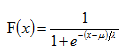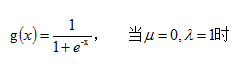2、在二分类问题中，为什么弃用传统的线性回归模型，改用逻辑斯蒂回归？
线性回归用于二分类时，首先想到下面这种形式，p是属于类别的概率：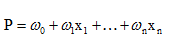但是这时存在的问题是：
1）等式两边的取值范围不同，右边是负无穷到正无穷，左边是[0,1]，这个分类模型的存在问题
2）实际中的很多问题，都是当x很小或很大时，对于因变量P的影响很小，当x达到中间某个阈值时，影响很大。即实际中很多问题，概率P与自变量并不是直线关系。
所以，上面这分类模型需要修整，怎么修正呢？统计学家们找到的一种方法是通过logit变换对因变量加以变换，具体如下：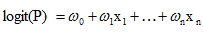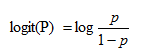从而，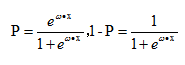这里的P完全解决了上面的两个问题。
3、逻辑回归模型的求解过程？
1）求解方式
逻辑回归中，Y服从二项分布，误差服从二项分布，而非高斯分布，所以不能用最小二乘进行模型参数估计，可以用极大似然估计来进行参数估计。
2）似然函数、目标函数
严谨一点的公式如下：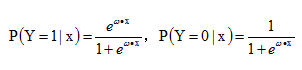似然函数如下：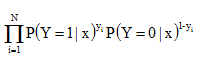对数似然函数，优化目标函数如下：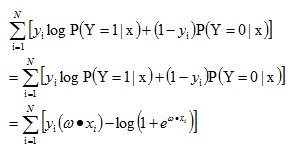整个逻辑回归问题就转化为求解目标函数，即对数似然函数的极大值的问题，即最优化问题，可采用梯度下降法、拟牛顿法等等。

4、实际应用逻辑回归时数据预处理的经验总结，但经验有限，如果有哪位网友这块经验丰富，忘指教，先谢过
1）枚举型的特征直接进行binary
2）数值型特征，可以：标准化、根据分布进行binary
3）进行pairwise
5、为什么我们在实际中，经典线性模型的优化目标函数是最小二乘，而逻辑回归则是似然函数
下面公式直接从Ng notes里面复制过来。
1） 经典线性模型的满足下面等式：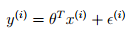这里有个假设，即最后这个误差扰动项独立同分布于均值为0的正态分布，即：从而：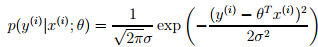由于有上面的假设，从而就有下面的似然函数：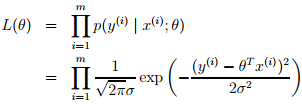从而这线性回归的问题就可转化为最大化下面的对数似然估计，由于下面公式前面的项是常数，所以这个问题等价于最小化下面等式中的最后一项，即least mean squares。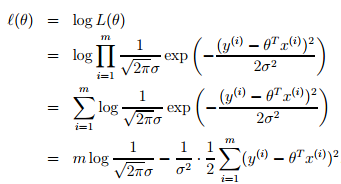2）逻辑斯蒂回归中，因变量y不再是连续的变量，而是二值的{0,1}，中间用到logit变换，将连续性的y值通过此变换映射到比较合理的0~1区间。在广义线性回归用于分类问题中，也有一个假设（对应于上面回归问题中误差项独立同分布于正态分布），其中h(x)是logistic function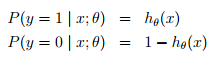即，给定x和参数，y服从二项分布，上面回归问题中，给定x和参数，y服从正态分布。从而。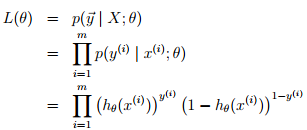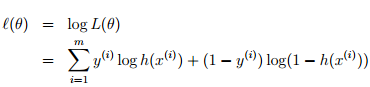问题不同（一个是分类、一个是回归）对应假设也就不同，决定了logistic regression问题最优化目标函数是上面这项，而非回归问题中的均方误差LMS。
6、从最根本的广义线性模型角度，导出经典线性模型以及逻辑回归
1）指数家族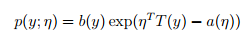当固定T时，这个分布属于指数家族中的哪种分布就由a和b两个函数决定。下面这种是伯努利分布，对应于逻辑回归问题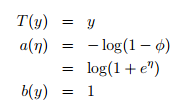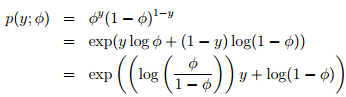注：从上面可知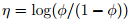，从而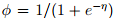，在后面用GLM导logistic regression的时候会用到这个sigmoid函数。

下面这种是高斯分布，对应于经典线性回归问题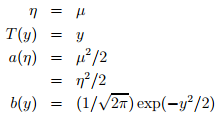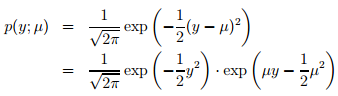2）GLM（广义线性模型）
指数家族的问题可以通过广义线性模型来解决。如何构建GLM呢？在给定x和参数后，y的条件概率p(y|x,θ) 需要满足下面三个假设：
assum1)      y | x; θ ∼ ExponentialFamily(η).
assum2)      h(x) = E[y|x]. 即给定x，目标是预测T(y)的期望，通常问题中T(y)=y
assum3)       η = θTx，即η和x之间是线性的
3）经典线性回归、逻辑回归
经典线性回归：预测值y是连续的，假设给定x和参数，y的概率分布服从高斯分布（对应构建GLM的第一条假设）。由上面高斯分布和指数家族分布的对应关系可知，η=µ，根据构建GLM的第2、3条假设可将model表示成：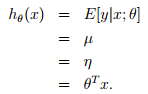逻辑回归：以二分类为例，预测值y是二值的{1,0}，假设给定x和参数，y的概率分布服从伯努利分布（对应构建GLM的第一条假设）。由上面高斯分布和指数家族分布的对应关系可知，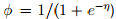，根据构建GLM的第2、3条假设可model表示成：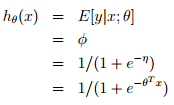可以从GLM这种角度理解为什么logistic regression的公式是这个形式~

参考资料：
 NG的lecture notes，http://cs229.stanford.edu/notes/cs229-notes1.pdf
 其他网络资源
展开全文逻辑回归
• 中科大对数线性模型读书笔记，数据预处理的使用场景。
• 对数线性模型有：最大熵模型和逻辑斯谛回归。 特征和指示特征 对数线性模型的一般形式 [概率图模型原理与技术] 某小皮 对数线性模型的不同形式 因子图 将因子转换到对数空间，成为对数线性模型...
http://blog.csdn.net/pipisorry/article/details/52788947
对数线性模型log linear model
对数线性模型有：最大熵模型和逻辑斯谛回归。
特征和指示特征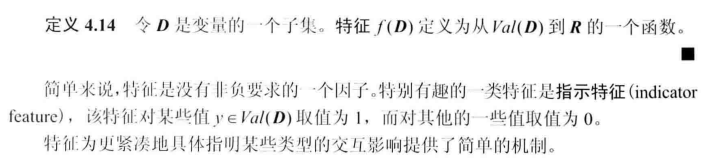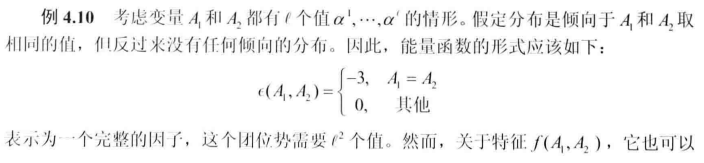对数线性模型的一般形式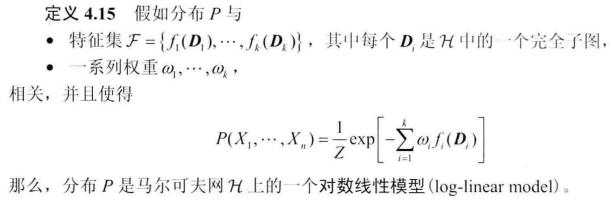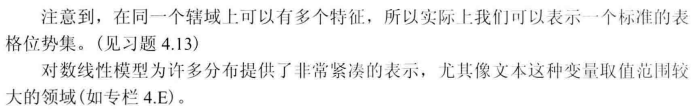[概率图模型原理与技术]
某小皮

对数线性模型的不同形式
因子图
将因子转换到对数空间，成为对数线性模型。
[PGM：无向图模型：马尔可夫网 ：对数线性模型]

最大熵模型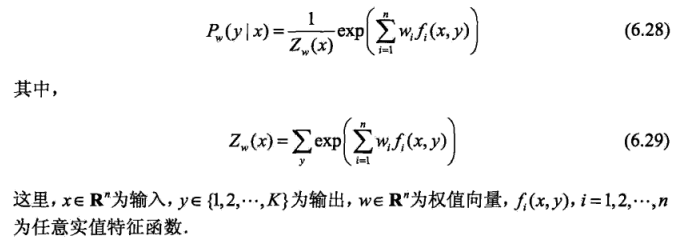[最大熵模型The Maximum Entropy：模型] [最大熵模型：学习]

逻辑斯谛回归Logistic Regression
多类分类的LR模型生成的推导：（两类分类更简单，直接类比嘛）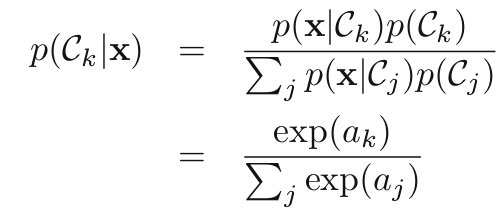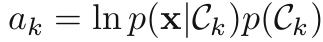lz：这里ak是对数表示的，而给定类条件概率密度p(x|ck)如高斯分布时，ak是通常是线性表示的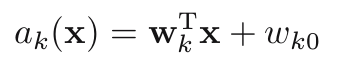，所以才叫对数线性模型吧。
因为ak通常可以使用线性表示，所以多类LR模型使用判别式直接定义成：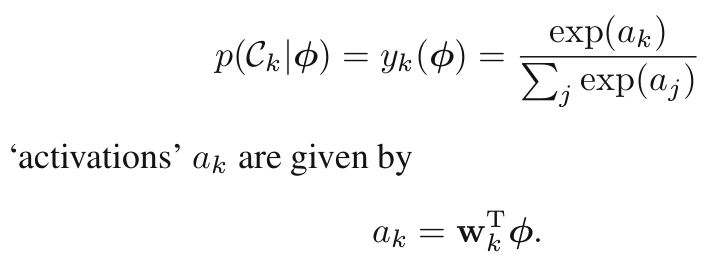lz: 就是把一般形式中的feature特征fi(Di)定义为ak了。
LR模型的导出
lz也不知道LR模型怎么来的，不过lz总结了几种都可以解释的方面：
1 回归模型+logistic函数直接得到
2 最大熵模型的特例，即直接将特征f(x, y)设为X=x（即在所有X=x的值上搞一个权重w）。
3 广义线性模型导出[对数线性模型之一(逻辑回归), 广义线性模型学习总结]
4 生成式模型+高斯形式的类条件概率分布得到
from: http://blog.csdn.net/pipisorry/article/details/52788947
ref:

展开全文• 基于双对数模型的广州市居民用水需求弹性分析，毕志化，郭海华，1980~2010年，广州市的居民生活用水价格经过7次上调，由最初的0.12元/立方米上调到现在的1.32元/立方米，平均每年上涨8.32%。根据双对数�
• 行业分类-电子-一种忆感器对数模型等效电路.zip
• 行业分类-电子-关于忆感器对数模型等效电路的说明分析.rar
• 运用多项式分对数模型对所提取的时空数据进行统计分类分析，依托特征参数建立交通状态多项K一Logit指数模型。结合快速路匝道控制措施。采用vISsIM COM与VC++6.O为仿真平台，对实验数据进行仿真，结果表明：分对数...
• 粒度分析中混合φ正态模型与混合对数正态模型的关系，郑海龙，刘小军，本文研究了沉积物粒度分析中的混合φ正态模型与混合对数正态模型之间的关系。作者用概率论基础知识证明两种模型是一致的。根据这�
• 通过更精确的先验估计，利用重合度理论中的连续定理，研究了一类时滞种群模型的周期解，获得了这类模型存在正周期解的充分条件，所得结果推广了文中的有关结论，并使条件有所减弱。
• 错误指定对Weibull模型对数正态模型之间均值和选择的影响研究论文
• 该软件包将时间强度数据与对数正态灌注模型 [2,3] 拟合。 Run_logperf_fit.m 使模型适合样本数据，并可用作任意中断补充数据的模板。 参考：  魏等。 用超声诱导的微泡破坏作为恒定静脉输注对心肌血流进行...matlab
• 为克服传统对数图像处理模型在边缘检测中存在的边缘定位不准确、检测精度差等缺点，对其进行了改进，并采用实拍复杂背景条件下红外机场跑道进行了边缘检测试验，结果表明，改进后算法的目标边缘检测精度明显提高，...
• ## 对数几率回归模型

千次阅读 2018-11-18 20:02:14
1.对数几率回归 本质是一个神经元。 对数几率回归可以回答Yes-No类的问题。 logistic函数，亦称为sigmod函数。...对于多维数据，需要将他们合并为单个值，可利用前面的线性回归模型表达式。 -*-牢记：sigmod...
1.对数几率回归
本质是一个神经元。
对数几率回归可以回答Yes-No类的问题。
logistic函数，亦称为sigmod函数。 f(x)=1/(1+exp(-x))
sigmod函数是一个概率分布函数,即给定某个特定输入，该函数将计算输出为“success”的概率。
sigmod函数接收单个输入值。对于多维数据，需要将他们合并为单个值，可利用前面的线性回归模型表达式。

-*-牢记：sigmod函数的输出是一个概率值。
2.预测部份代码
#与对数几率回归相同的参数和变量初始化
W = tf.Variable(tf.zeros([5, 1]),name="weights")
b = tf.Variable(0, name="bias")

#之前的推断现在用于值的合并
def combine_inputs(X):
return tf.matul(X, W) + b

#新的推断值是将sigmod函数运用到前面的合并值的输出
def inference(X):
return tf.sigmod(combine_inputs(X))

3.损失函数 －＊－交叉熵的原理，详情见：https://blog.csdn.net/tsyccnh/article/details/79163834。
交叉熵可表示预测输出跟期望输出的接近程度，越接近，交叉熵越小。
（1）对于单分类问题 单分类问题：这里的单类别是指，每一张图像样本只能有一个类别，比如只能是狗或只能是猫，单类别的标签是one-hot。  loss = -[p1log(q1) + p2log(q2) + … + pn*log(qn)] 其中pi为期望输出概率，qi为预测输出概率，n为1个样本可能的输出数目。
（2）对于多分类问题 多分类问题：这里的多类别是指，每一张图像样本可以有多个类别，比如同时包含一只猫和一只狗 ，和单分类问题的标签不同，多分类的标签是n-hot。
展开全文• 对数线性模型包括逻辑回归、最大熵模型和条件随机场等 1、模型 条件概率分布（对数线性模型、概率模型）、判别模型 逻辑回归： 概率分布可由广义线性模型推导得到 多分类时，分子部分为：第k类exp(-wk*x+b)，...
• 将变量对数转换后，如何解释这个变量的影响呢？ 答： 对数转换后可分为双对数和半对数模型，系数解释如下： 原地址：https://www.zhihu.com/question/379297282
• 从线性到非线性模型 1、线性回归，岭回归，Lasso回归，局部加权线性回归 2、logistic回归，softmax回归，最大熵模型 3、广义线性模型 4、Fisher线性判别和线性感知机 5、三层...
• 对数图像处理新模型及其应用研究 西安电子科技大学 硕士毕业论文 详细介绍了 对数图像处理模型( LIS )及其应用研究
• Virasoro Kac模块最初是作为表示形式间接引入的，其特征出现在使用Temperley-Lieb代数描述的对数最小模型中某些传递矩阵的连续标度限制中。 晶格转移算子包括使用Wenzl-Jones投影仪的边界接缝。 如果投影仪是单个...
• MIT自然语言处理第五讲：最大熵和对数线性模型（第一部分） 自然语言处理：最大熵和对数线性模型 Natural Language Processing: Maximum Entropy and Log-linear Models  作者：Regina Barzilay...MIT 自然语言处理 NLP...